# Layering Materials

You can layer materials with a high degree of control over each layer.

The area below the Material name lists the layers in the selected material. When you select a layer from the list, the corresponding layer type (Basic, Metal, etc.) is highlighted.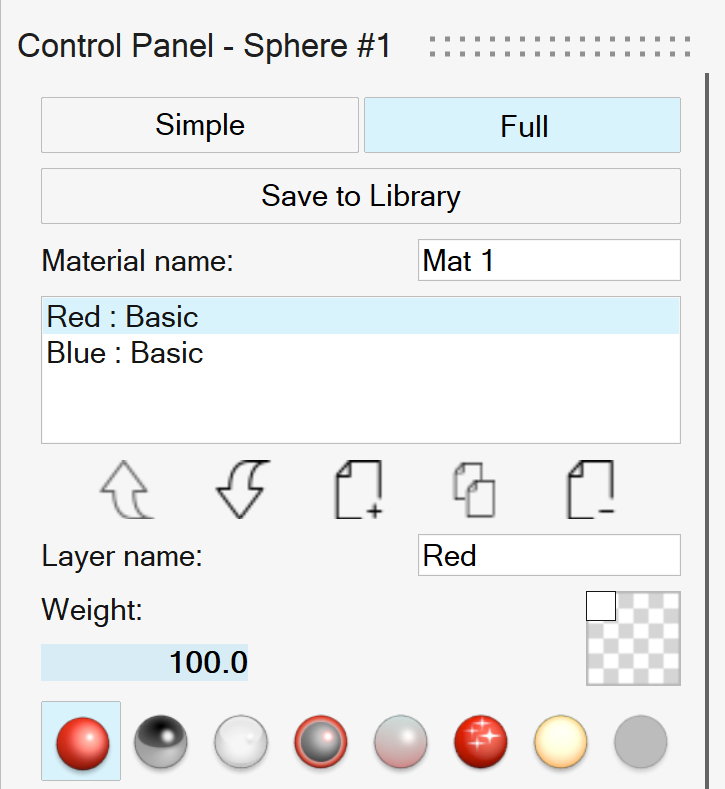To Do this Note
Add a layer Click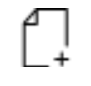.
Delete a layer Select the layer, and then click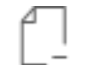.
Move a layer up Select the layer, and then click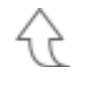.
Move a layer down Select the layer, and then click.
Duplicate a layer Select the layer, and then click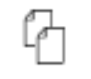. The copy will be pasted as the topmost layer.
Name a layer Select the layer, and then enter a Layer name.
Change the layer's weight Select the layer, and then enter a Weight from 0 to 100. By default, the weight is 100. Layer weight influences the material from top to bottom.
Change the layer type Select the layer, and then select one of the following:
• Basic• Metal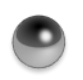• Glass/Gems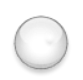• Coating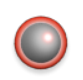• Subsurface Scattering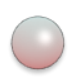• Metallic Flake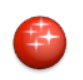• Emitter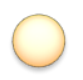• Shadow Catcher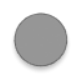## Weight and Stacking Order

The weight and stacking order of the layers play a significant role in the final look of the material.

In the example below, there are two basic layers named Red and Blue.

### Weight

Layer weight influences the material from top to bottom. If the topmost layer has a weight of 100%, then any layer underneath will not be considered by the engine.

Here, the blue basic layer weight is set to 100%. Thus, we cannot see the red basic layer underneath.

All layers are normalized according to their sum, so that each layer's reflectance is modified by a relative percentage. Without any weights, the layers are again normalized to reflect the same weights.

The weight of the coating layer modifies the percentage of light allowed to penetrate the layers underneath.

### Stacking Order

The stacking order of the layers can significantly change the final appearance of the material. In the example, changing the stacking order changes the color of the material.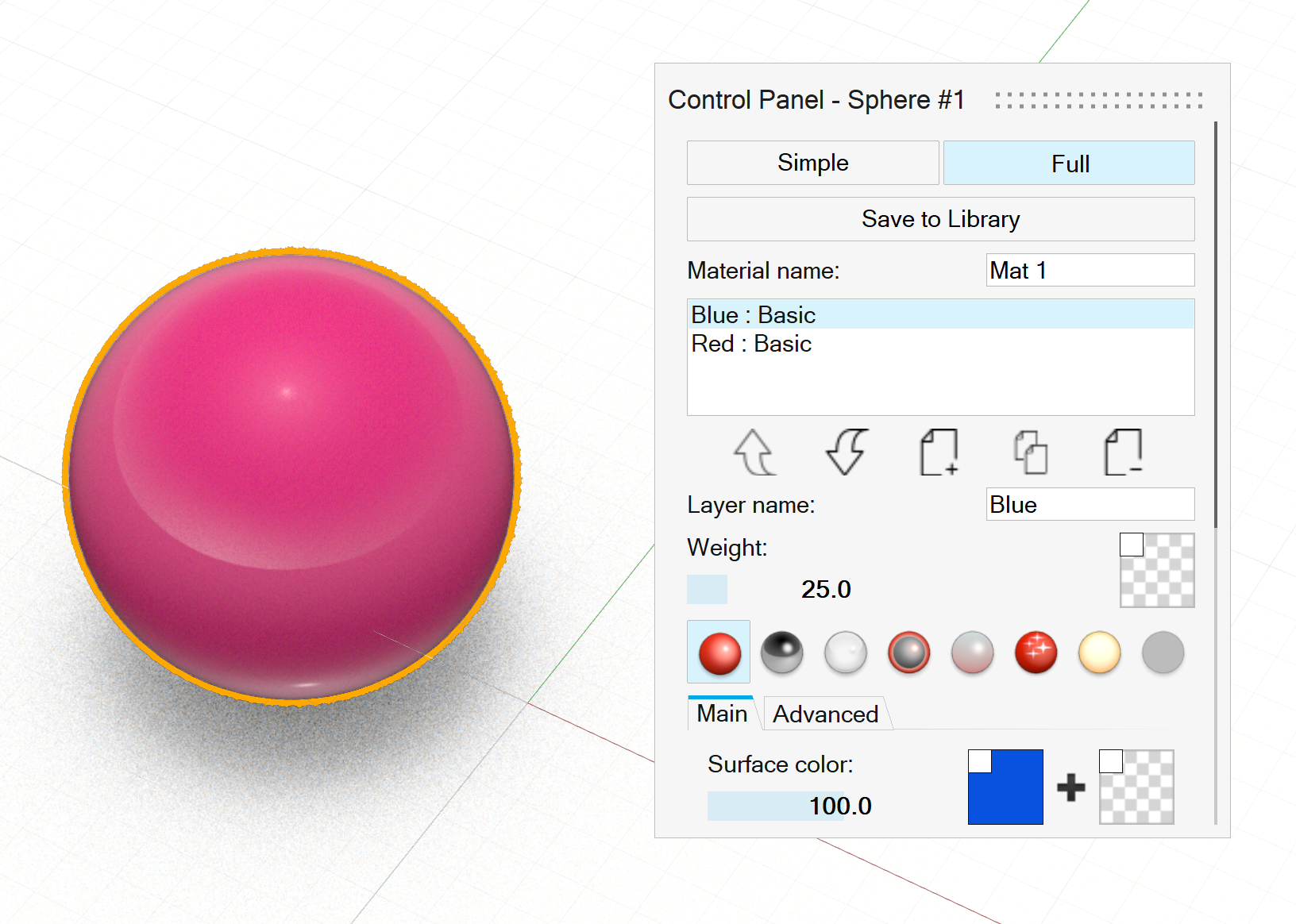Figure 1. Blue on top of Red; Blue weight = 25%, Red weight = 100%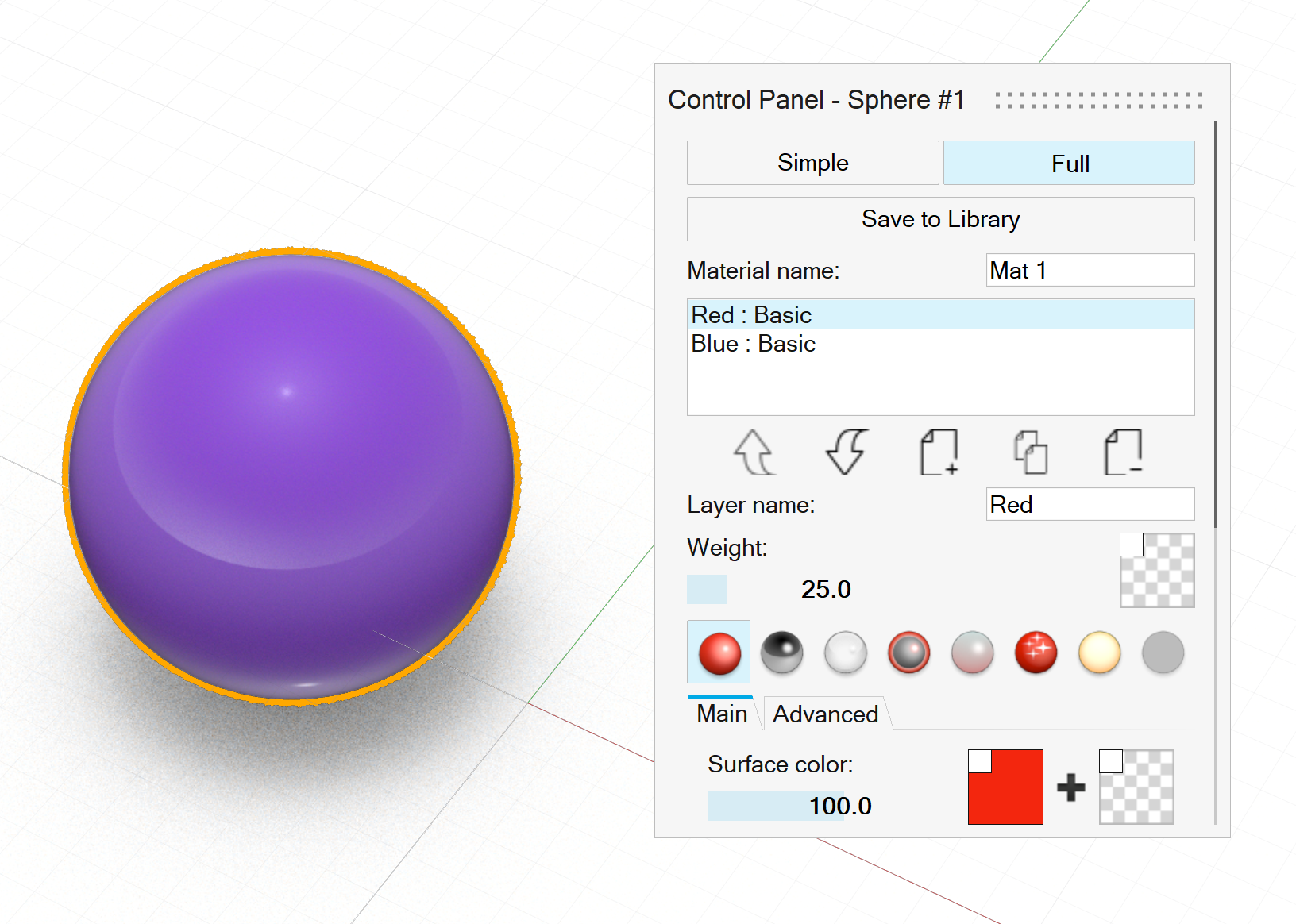Figure 2. Red on top of Blue; Red weight = 25%, Blue weight = 100%